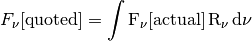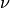# Convolving models with new filters¶

## Preparing filters¶

This section describes how to convolve model packages with new filters. As described in the appendix Robitaille et al. (2007), there are a number of ways of defining filters/responses - for example one can define them as the fraction of photons that pass through the system, or the fraction of the energy that passes through the system. One also needs to understand what assumptions are being made to be able to quote a monochromatic flux for a broadband flux (for example, MIPS 24 micron fluxes typically assume that the underlying spectrum is a 10,000K blackbody).

The details of working out these assumptions is left to the user, and the Filter class used in the SED fitter requires that the user has already transformed and normalized the filter, such that the convolution can be done using the simple expression:The filter class is imported using:

from sedfitter.filter import Filter


and should be instantiated as follows:

f = Filter()
f.name = ...
f.central_wavelength = ...
f.nu = ...
f.response = ...


for example:

f = Filter()
f.name = "NEW_FILT"
f.central_wavelength = 130. * u.micron
f.nu = np.logspace(12., 13., 100) * u.Hz
f.response = np.exp(-(np.log10(f.nu.value) - 12.5)**2 / (2. * 0.1)**2)


The filter can then be normalized (so that the integral overis 1) using:

f.normalize()


Once you have set up and normalized the filters, you are now ready to use the convolution functions.

Assuming that you have a model directory from Available Model Packages or Creating model packages, and filters prepared as described above, you can now use the convolve_model_dir() function to create the required convolved flux files. This function is used as:

from sedfitter.convolve import convolve_model_dir
convolve_model_dir(model_dir, filters)


where model_dir is a string containing the path to the models directory, and filters is a list of Filter instances for which you want to compute the convolved fluxes. For example, using the filter object from above:

convolve_model_dir('models_r06', [f])


This will then create new files in models_r06/convolved/, and you will then be able to use the filters for any further fitting.

## Monochromatic ‘convolution’¶

In addition to conlving models with the SEDs, it is also possible to pretend that each wavelength for which the SEDs are defined can be associated with a delta function filter, and create a convolved flux file for each of these wavelengths. This can be done using:

from sedfitter.convolve import convolve_model_dir_monochromatic
filters = convolve_model_dir_monochromatic(model_dir)


This will return a table containing details about the ‘filters’ that have been created.